{ "cells": [ { "cell_type": "markdown", "metadata": {}, "source": [ "## Common plotting pitfalls that get worse with large data\n", "\n", "When working with large datasets, visualizations are often the only way available to understand the properties of that dataset -- there are simply too many data points to examine each one! Thus it is very important to be aware of some common plotting problems that are minor inconveniences with small datasets but very serious problems with larger ones.\n", "\n", "We'll cover:\n", "\n", "1. [Overplotting](#1.-Overplotting)\n", "2. [Oversaturation](#2.-Oversaturation)\n", "3. [Undersampling](#3.-Undersampling)\n", "4. [Undersaturation](#4.-Undersaturation)\n", "5. [Underutilized range](#5.-Underutilized-range)\n", "6. [Nonuniform colormapping](#6.-Nonuniform-colormapping)\n", "\n", "You can [skip to the end](#Summary) if you just want to see an illustration of these problems.\n", "\n", "This notebook requires [HoloViews](http://holoviews.org), [colorcet](https://github.com/bokeh/colorcet), and matplotlib, and optionally scikit-image, which can be installed with:\n", "\n", "```\n", "conda install -c bokeh -c ioam holoviews colorcet matplotlib scikit-image\n", "```\n", "\n", "We'll first load the plotting libraries and set up some defaults:" ] }, { "cell_type": "code", "execution_count": 1, "metadata": {}, "outputs": [ { "data": { "text/html": [ "\n", "\n", "\n", "\n", "\n", "\n", "\n", "\n", "\n", "
\n", "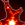\n", "\n", "\n", "
\n" ], "text/plain": [ "" ] }, "metadata": {}, "output_type": "display_data" }, { "name": "stderr", "output_type": "stream", "text": [ "/Users/jbednar/anaconda/envs/ds-test/lib/python3.6/site-packages/odo/backends/pandas.py:94: FutureWarning: pandas.tslib is deprecated and will be removed in a future version.\n", "You can access NaTType as type(pandas.NaT)\n", " @convert.register((pd.Timestamp, pd.Timedelta), (pd.tslib.NaTType, type(None)))\n" ] } ], "source": [ "import numpy as np\n", "np.random.seed(42)\n", "\n", "import holoviews as hv\n", "hv.notebook_extension('matplotlib')\n", "\n", "%opts Points [color_index=2] (cmap=\"bwr\" edgecolors='k' s=50 alpha=1.0)\n", "%opts Scatter3D [color_index=3 fig_size=250] (cmap='bwr' edgecolor='k' s=50 alpha=1.0)\n", "%opts Image (cmap=\"gray_r\") {+axiswise}\n", "%opts RGB [bgcolor=\"black\" show_grid=False]\n", "\n", "import holoviews.plotting.mpl\n", "holoviews.plotting.mpl.MPLPlot.fig_alpha = 0\n", "holoviews.plotting.mpl.ElementPlot.bgcolor = 'white'\n", "\n", "from holoviews.operation.datashader import datashade\n", "from colorcet import fire\n", "datashade.cmap=fire[50:]" ] }, { "cell_type": "markdown", "metadata": {}, "source": [ "### 1. Overplotting\n", "\n", "Let's consider plotting some 2D data points that come from two separate categories, here plotted as blue and red in **A** and **B** below. When the two categories are overlaid, the appearance of the result can be very different depending on which one is plotted first:" ] }, { "cell_type": "code", "execution_count": 2, "metadata": {}, "outputs": [ { "data": { "text/html": [ "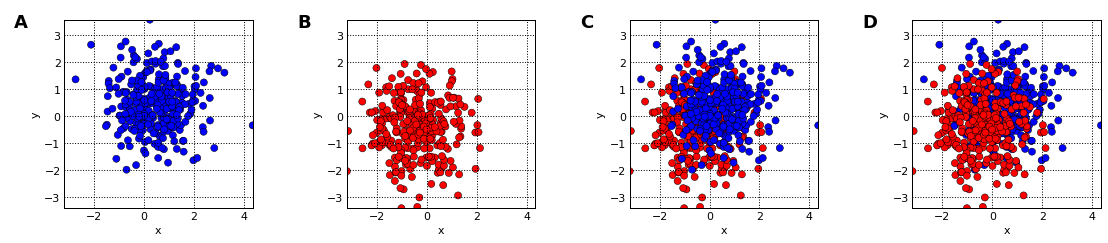" ], "text/plain": [ ":Layout\n", " .Points.I :Points [x,y] (c)\n", " .Points.II :Points [x,y] (c)\n", " .Overlay.I :Overlay\n", " .Points.I :Points [x,y] (c)\n", " .Points.II :Points [x,y] (c)\n", " .Overlay.II :Overlay\n", " .Points.I :Points [x,y] (c)\n", " .Points.II :Points [x,y] (c)" ] }, "execution_count": 2, "metadata": {}, "output_type": "execute_result" } ], "source": [ "def blues_reds(offset=0.5,pts=300):\n", " blues = (np.random.normal( offset,size=pts), np.random.normal( offset,size=pts), -1*np.ones((pts)))\n", " reds = (np.random.normal(-offset,size=pts), np.random.normal(-offset,size=pts), 1*np.ones((pts)))\n", " return hv.Points(blues, vdims=['c']), hv.Points(reds, vdims=['c'])\n", "\n", "blues,reds = blues_reds()\n", "blues + reds + reds*blues + blues*reds" ] }, { "cell_type": "markdown", "metadata": {}, "source": [ "Plots **C** and **D** shown the same distribution of points, yet they give a very different impression of which category is more common, which can lead to incorrect decisions based on this data. Of course, both are equally common in this case. The cause for this problem is simply occlusion:" ] }, { "cell_type": "code", "execution_count": 3, "metadata": {}, "outputs": [ { "data": { "text/html": [ "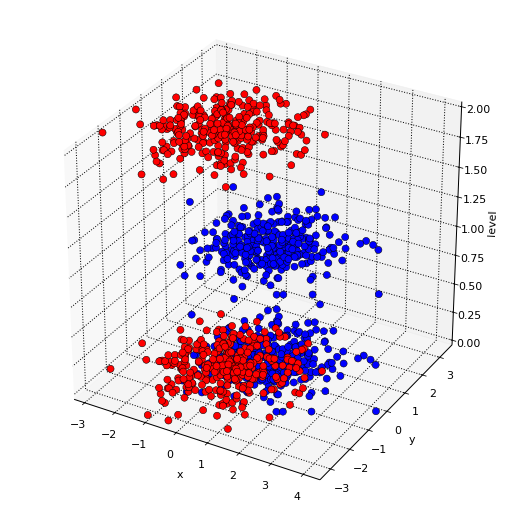" ], "text/plain": [ ":Scatter3D [x,y,level] (c)" ] }, "execution_count": 3, "metadata": {}, "output_type": "execute_result" } ], "source": [ "hmap = hv.HoloMap({0:blues,0.000001:reds,1:blues,2:reds}, key_dimensions=['level'])\n", "hv.Scatter3D(hmap.table(), kdims=['x','y','level'], vdims=['c'])" ] }, { "cell_type": "markdown", "metadata": {}, "source": [ "Occlusion of data by other data is called **overplotting** or **overdrawing**, and it occurs whenever a datapoint or curve is plotted on top of another datapoint or curve, obscuring it. It's thus a problem not just for scatterplots, as here, but for curve plots, 3D surface plots, 3D bar graphs, and any other plot type where data can be obscured.\n", "\n", "\n", "### 2. Oversaturation\n", "\n", "You can reduce problems with overplotting by using transparency/opacity, via the alpha parameter provided to control opacity in most plotting programs. E.g. if alpha is 0.1, full color saturation will be achieved only when 10 points overlap, reducing the effects of plot ordering but making it harder to see individual points:" ] }, { "cell_type": "code", "execution_count": 4, "metadata": {}, "outputs": [ { "data": { "text/html": [ "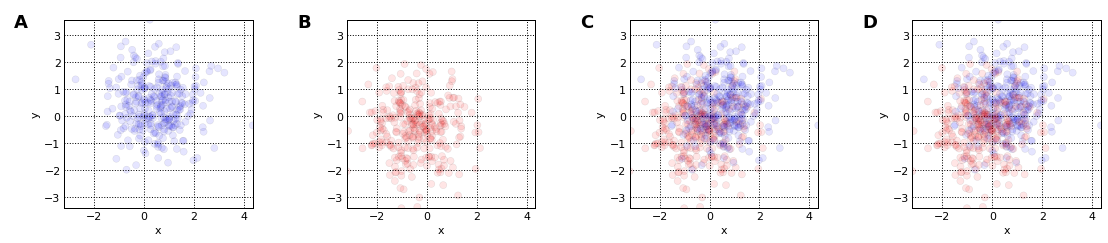" ], "text/plain": [ ":Layout\n", " .Points.I :Points [x,y] (c)\n", " .Points.II :Points [x,y] (c)\n", " .Overlay.I :Overlay\n", " .Points.I :Points [x,y] (c)\n", " .Points.II :Points [x,y] (c)\n", " .Overlay.II :Overlay\n", " .Points.I :Points [x,y] (c)\n", " .Points.II :Points [x,y] (c)" ] }, "execution_count": 4, "metadata": {}, "output_type": "execute_result" } ], "source": [ "%%opts Points (s=50 alpha=0.1)\n", "blues + reds + reds*blues + blues*reds" ] }, { "cell_type": "markdown", "metadata": {}, "source": [ "Here **C** and **D** look very similar (as they should, since the distributions are identical), but there are still a few locations with **oversaturation**, a problem that will occur when more than 10 points overlap. In this example the oversaturated points are located near the middle of the plot, but the only way to know whether they are there would be to plot both versions and compare, or to examine the pixel values to see if any have reached full saturation (a necessary but not sufficient condition for oversaturation). Locations where saturation has been reached have problems similar to overplotting, because only the last 10 points plotted will affect the final color (for alpha of 0.1).\n", "\n", "Worse, even if one has set the alpha value to approximately or usually avoid oversaturation, as in the plot above, the correct value depends on the dataset. If there are more points overlapping in that particular region, a manually adjusted alpha setting that worked well for a previous dataset will systematically misrepresent the new dataset:" ] }, { "cell_type": "code", "execution_count": 5, "metadata": {}, "outputs": [ { "data": { "text/html": [ "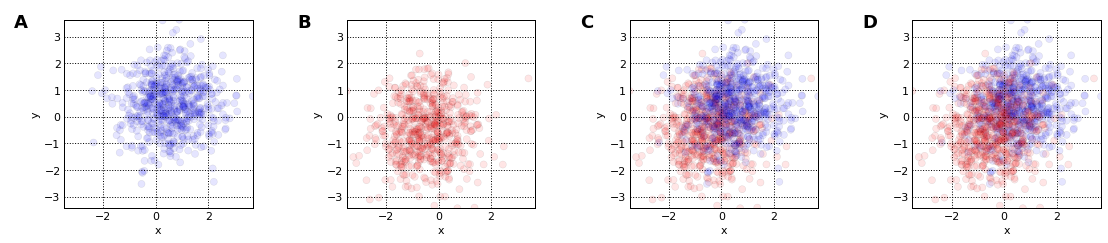" ], "text/plain": [ ":Layout\n", " .Points.I :Points [x,y] (c)\n", " .Points.II :Points [x,y] (c)\n", " .Overlay.I :Overlay\n", " .Points.I :Points [x,y] (c)\n", " .Points.II :Points [x,y] (c)\n", " .Overlay.II :Overlay\n", " .Points.I :Points [x,y] (c)\n", " .Points.II :Points [x,y] (c)" ] }, "execution_count": 5, "metadata": {}, "output_type": "execute_result" } ], "source": [ "%%opts Points (alpha=0.1)\n", "blues,reds = blues_reds(pts=600)\n", "blues + reds + reds*blues + blues*reds" ] }, { "cell_type": "markdown", "metadata": {}, "source": [ "Here **C** and **D** again look qualitatively different, yet still represent the same distributions. Since we're assuming that the point of the visualization is to reveal the underlying dataset, having to tune visualization parameters manually based on the properties of the dataset itself is a serious problem.\n", "\n", "To make it even more complicated, the correct alpha also depends on the dot size, because smaller dots have less overlap for the same dataset. With smaller dots, **C** and **D** look more similar, but the color of the dots is now difficult to see in all cases because the dots are too transparent for this size:" ] }, { "cell_type": "code", "execution_count": 6, "metadata": {}, "outputs": [ { "data": { "text/html": [ "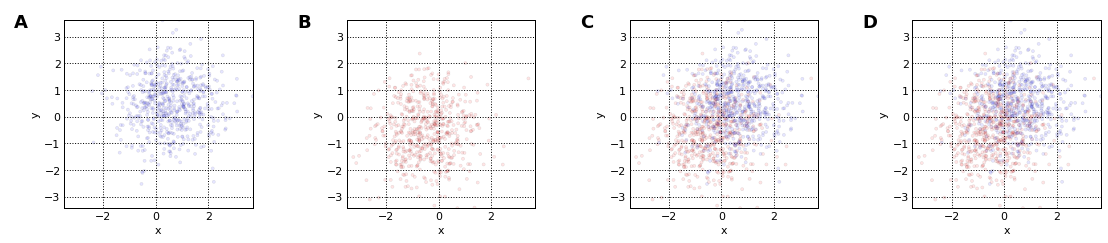" ], "text/plain": [ ":Layout\n", " .Points.I :Points [x,y] (c)\n", " .Points.II :Points [x,y] (c)\n", " .Overlay.I :Overlay\n", " .Points.I :Points [x,y] (c)\n", " .Points.II :Points [x,y] (c)\n", " .Overlay.II :Overlay\n", " .Points.I :Points [x,y] (c)\n", " .Points.II :Points [x,y] (c)" ] }, "execution_count": 6, "metadata": {}, "output_type": "execute_result" } ], "source": [ "%%opts Points (s=10 alpha=0.1 edgecolor=None)\n", "blues + reds + reds*blues + blues*reds" ] }, { "cell_type": "markdown", "metadata": {}, "source": [ "As you can see, it is very difficult to find settings for the dotsize and alpha parameters that correctly reveal the data, even for relatively small and obvious datasets like these. With larger datasets with unknown contents, it is difficult to detect that such problems are occuring, leading to false conclusions based on inappropriately visualized data.\n", "\n", "### 3. Undersampling\n", "\n", "With a single category instead of the multiple categories shown above, oversaturation simply obscures spatial differences in density. For instance, 10, 20, and 2000 single-category points overlapping will all look the same visually, for alpha=0.1. Let's again consider an example that has a sum of two normal distributions slightly offset from one another, but no longer using color to separate them into categories:" ] }, { "cell_type": "code", "execution_count": 7, "metadata": {}, "outputs": [ { "data": { "text/html": [ "